# 9.2 Arithmetic sequences  (Page 6/8)

 Page 6 / 8

For the following exercises, use the explicit formula to write the first five terms of the arithmetic sequence.

${a}_{n}=24-4n$

First five terms: $20,16,12,8,4.$

${a}_{n}=\frac{1}{2}n-\frac{1}{2}$

For the following exercises, write an explicit formula for each arithmetic sequence.

${a}_{n}=\left\{3,5,7,...\right\}$

${a}_{n}=1+2n$

${a}_{n}=\left\{32,24,16,...\right\}$

${a}_{n}=-105+100n$

${a}_{n}=1.8n$

${a}_{n}=\left\{-18.1,-16.2,-14.3,...\right\}$

${a}_{n}=\left\{15.8,18.5,21.2,...\right\}$

${a}_{n}=13.1+2.7n$

${a}_{n}=\left\{0,\frac{1}{3},\frac{2}{3},...\right\}$

${a}_{n}=\frac{1}{3}n-\frac{1}{3}$

${a}_{n}=\left\{-5,-\frac{10}{3},-\frac{5}{3},\dots \right\}$

For the following exercises, find the number of terms in the given finite arithmetic sequence.

There are 10 terms in the sequence.

${a}_{n}=\left\{1.2,1.4,1.6,...,3.8\right\}$

${a}_{n}=\left\{\frac{1}{2},2,\frac{7}{2},...,8\right\}$

There are 6 terms in the sequence.

## Graphical

For the following exercises, determine whether the graph shown represents an arithmetic sequence.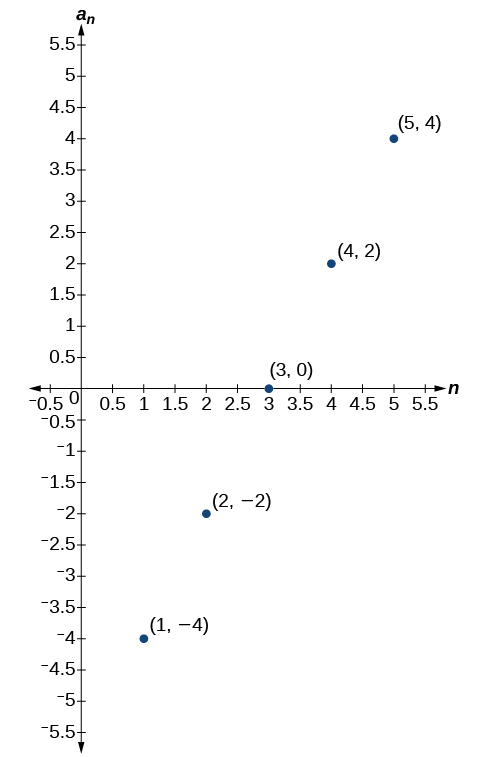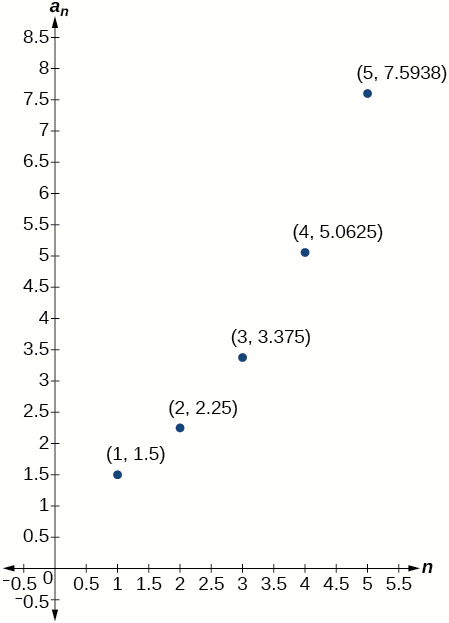The graph does not represent an arithmetic sequence.

For the following exercises, use the information provided to graph the first 5 terms of the arithmetic sequence.

${a}_{1}=0,d=4$

${a}_{1}=9;{a}_{n}={a}_{n-1}-10$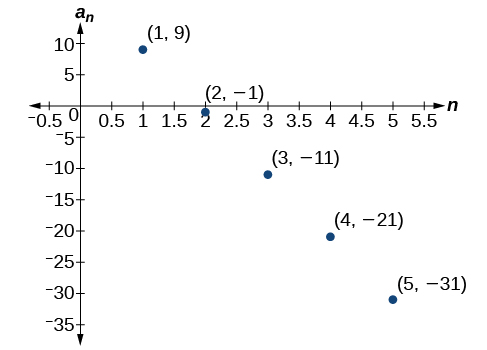${a}_{n}=-12+5n$

## Technology

For the following exercises, follow the steps to work with the arithmetic sequence ${a}_{n}=3n-2$ using a graphing calculator:

• Press [MODE]
• Select SEQ in the fourth line
• Select DOT in the fifth line
• Press [ENTER]
• Press [Y=]
• $n\text{Min}\text{\hspace{0.17em}}$ is the first counting number for the sequence. Set $\text{\hspace{0.17em}}n\text{Min}=1$
• $u\left(n\right)\text{\hspace{0.17em}}$ is the pattern for the sequence. Set $\text{\hspace{0.17em}}u\left(n\right)=3n-2$
• $u\left(n\text{Min)}\text{\hspace{0.17em}}$ is the first number in the sequence. Set $\text{\hspace{0.17em}}u\left(n\text{Min)}=1$
• Press [2ND] then [WINDOW] to go to TBLSET
• Set $\text{\hspace{0.17em}}\text{TblStart}=1$
• Set $\text{\hspace{0.17em}}\Delta \text{Tbl}=1$
• Set Indpnt: Auto and Depend: Auto
• Press [2ND] then [GRAPH] to go to the TABLE

What are the first seven terms shown in the column with the heading $u\left(n\right)\text{?}$

$1,4,7,10,13,16,19$

Use the scroll-down arrow to scroll to $n=50.$ What value is given for $u\left(n\right)\text{?}$

Press [WINDOW] . Set $\text{\hspace{0.17em}}n\text{Min}=1,n\text{Max}=5,x\text{Min}=0,x\text{Max}=6,y\text{Min}=-1,\text{\hspace{0.17em}}$ and $\text{\hspace{0.17em}}y\text{Max}=14.\text{\hspace{0.17em}}$ Then press [GRAPH] . Graph the sequence as it appears on the graphing calculator.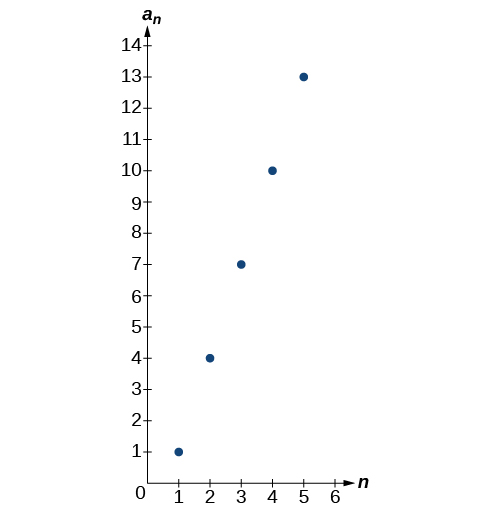For the following exercises, follow the steps given above to work with the arithmetic sequence ${a}_{n}=\frac{1}{2}n+5$ using a graphing calculator.

What are the first seven terms shown in the column with the heading $\text{\hspace{0.17em}}u\left(n\right)\text{\hspace{0.17em}}$ in the TABLE feature?

Graph the sequence as it appears on the graphing calculator. Be sure to adjust the WINDOW settings as needed.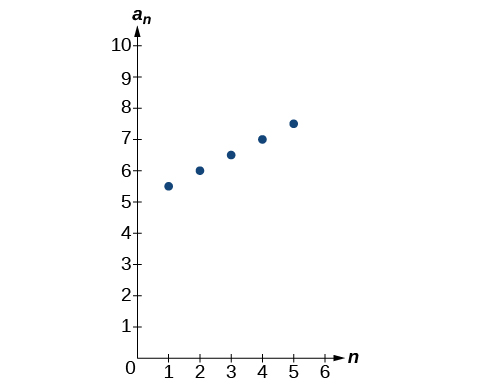## Extensions

Give two examples of arithmetic sequences whose 4 th terms are $9.$

Give two examples of arithmetic sequences whose 10 th terms are $206.$

Answers will vary. Examples: ${a}_{n}=20.6n$ and ${a}_{n}=2+20.4\mathrm{n.}$

Find the 5 th term of the arithmetic sequence $\left\{9b,5b,b,\dots \right\}.$

Find the 11 th term of the arithmetic sequence $\left\{3a-2b,a+2b,-a+6b\dots \right\}.$

${a}_{11}=-17a+38b$

At which term does the sequence $\left\{5.4,14.5,23.6,...\right\}$ exceed 151?

At which term does the sequence $\left\{\frac{17}{3},\frac{31}{6},\frac{14}{3},...\right\}$ begin to have negative values?

The sequence begins to have negative values at the 13 th term, ${a}_{13}=-\frac{1}{3}$

For which terms does the finite arithmetic sequence $\left\{\frac{5}{2},\frac{19}{8},\frac{9}{4},...,\frac{1}{8}\right\}$ have integer values?

Write an arithmetic sequence using a recursive formula. Show the first 4 terms, and then find the 31 st term.

Answers will vary. Check to see that the sequence is arithmetic. Example: Recursive formula: ${a}_{1}=3,{a}_{n}={a}_{n-1}-3.$ First 4 terms: $\begin{array}{ll}3,0,-3,-6\hfill & {a}_{31}=-87\hfill \end{array}$

Write an arithmetic sequence using an explicit formula. Show the first 4 terms, and then find the 28 th term.

show that the set of all natural number form semi group under the composition of addition
explain and give four Example hyperbolic function
_3_2_1
felecia
⅗ ⅔½
felecia
_½+⅔-¾
felecia
The denominator of a certain fraction is 9 more than the numerator. If 6 is added to both terms of the fraction, the value of the fraction becomes 2/3. Find the original fraction. 2. The sum of the least and greatest of 3 consecutive integers is 60. What are the valu
1. x + 6 2 -------------- = _ x + 9 + 6 3 x + 6 3 ----------- x -- (cross multiply) x + 15 2 3(x + 6) = 2(x + 15) 3x + 18 = 2x + 30 (-2x from both) x + 18 = 30 (-18 from both) x = 12 Test: 12 + 6 18 2 -------------- = --- = --- 12 + 9 + 6 27 3
Pawel
2. (x) + (x + 2) = 60 2x + 2 = 60 2x = 58 x = 29 29, 30, & 31
Pawel
ok
Ifeanyi
on number 2 question How did you got 2x +2
Ifeanyi
combine like terms. x + x + 2 is same as 2x + 2
Pawel
x*x=2
felecia
2+2x=
felecia
Mark and Don are planning to sell each of their marble collections at a garage sale. If Don has 1 more than 3 times the number of marbles Mark has, how many does each boy have to sell if the total number of marbles is 113?
Mark = x,. Don = 3x + 1 x + 3x + 1 = 113 4x = 112, x = 28 Mark = 28, Don = 85, 28 + 85 = 113
Pawel
how do I set up the problem?
what is a solution set?
Harshika
find the subring of gaussian integers?
Rofiqul
hello, I am happy to help!
Abdullahi
hi mam
Mark
find the value of 2x=32
divide by 2 on each side of the equal sign to solve for x
corri
X=16
Michael
Want to review on complex number 1.What are complex number 2.How to solve complex number problems.
Beyan
yes i wantt to review
Mark
use the y -intercept and slope to sketch the graph of the equation y=6x
how do we prove the quadratic formular
Darius
hello, if you have a question about Algebra 2. I may be able to help. I am an Algebra 2 Teacher
thank you help me with how to prove the quadratic equation
Seidu
may God blessed u for that. Please I want u to help me in sets.
Opoku
what is math number
4
Trista
x-2y+3z=-3 2x-y+z=7 -x+3y-z=6
can you teacch how to solve that🙏
Mark
Solve for the first variable in one of the equations, then substitute the result into the other equation. Point For: (6111,4111,−411)(6111,4111,-411) Equation Form: x=6111,y=4111,z=−411x=6111,y=4111,z=-411
Brenna
(61/11,41/11,−4/11)
Brenna
x=61/11 y=41/11 z=−4/11 x=61/11 y=41/11 z=-4/11
Brenna
Need help solving this problem (2/7)^-2
x+2y-z=7
Sidiki
what is the coefficient of -4×
-1
Shedrak

#### Get Jobilize Job Search Mobile App in your pocket Now!ByByBy OpenStaxBy Tess ArmstrongByBy Kimberly NicholsBy OpenStaxBy OpenStaxBy Katie MontroseBy JavaChamp TeamBy OpenStaxBy OpenStax